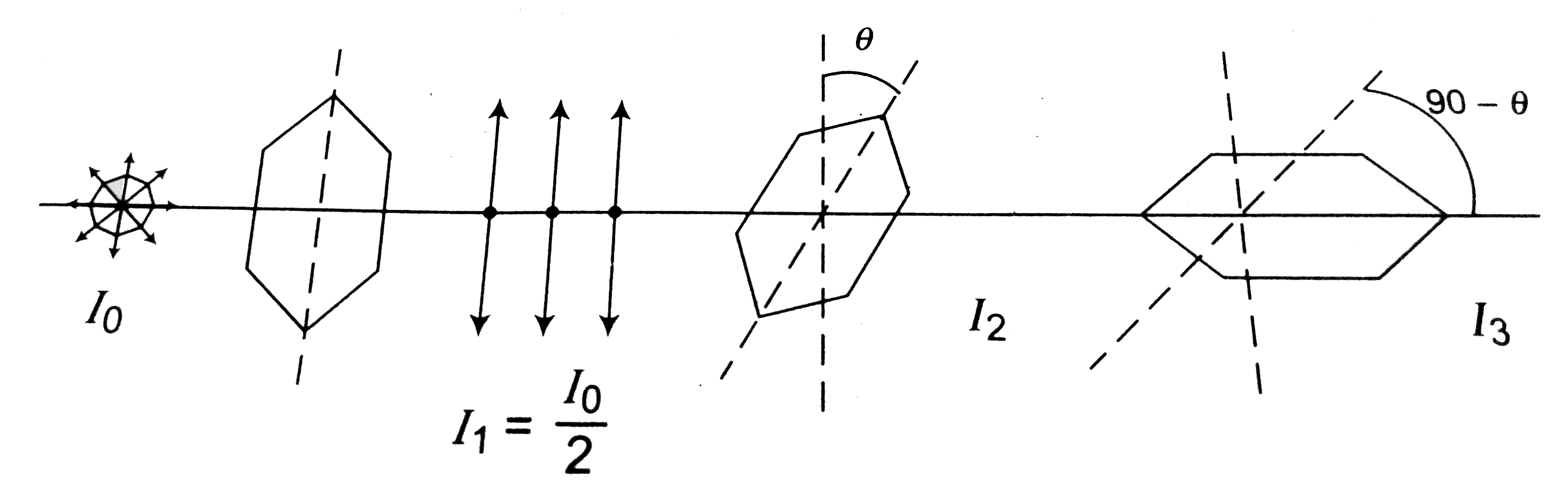# Two polaroids are placed in the path of unpolarized beam of intensity I_(0) such that no light is emitted from the second polarid. If a third polaro

45 views
in Physics
closed
Two polaroids are placed in the path of unpolarized beam of intensity I_(0) such that no light is emitted from the second polarid. If a third polaroid whose polarization axis makes an angle theta with the polarization axis of first polaroid, is placed between these two polariods then the intensity of light emerging from the last polaroid will be
A. (I_(0)/8) sin^(2) 2theta
B. (I_(0)/4) sin^(2) 2theta
C. (I_(0)/2) cos^(4) theta
D. I_(0) cos^(4) theta

by (84.1k points)
selectedI_(2)=I_(1) cos^(2) theta=I_(0)/2 cos^(2) theta
I_(3)=I_(2) cos^(2) (90-theta)=I_(0)/2 cos^(2) theta sin^(2) theta
=I_(0)/8(2 sin theta cos theta)^(2)=I_(0)/8(sin 2theta)^(2)